$\newcommand{\NN}{\mathbb{N}} \newcommand{\CC}{\mathbb{C}} \newcommand{\GG}{\mathbb{G}} \newcommand{\LL}{\mathbb{L}} \newcommand{\PP}{\mathbb{P}} \newcommand{\QQ}{\mathbb{Q}} \newcommand{\RR}{\mathbb{R}} \newcommand{\VV}{\mathbb{V}} \newcommand{\ZZ}{\mathbb{Z}} \newcommand{\FF}{\mathbb{F}} \newcommand{\KK}{\mathbb{K}} \newcommand{\UU}{\mathbb{U}} \newcommand{\EE}{\mathbb{E}} \newcommand{\Aa}{\mathcal{A}} \newcommand{\Bb}{\mathcal{B}} \newcommand{\Cc}{\mathcal{C}} \newcommand{\Dd}{\mathcal{D}} \newcommand{\Ee}{\mathcal{E}} \newcommand{\Ff}{\mathcal{F}} \newcommand{\Gg}{\mathcal{G}} \newcommand{\Hh}{\mathcal{H}} \newcommand{\Ii}{\mathcal{I}} \newcommand{\Jj}{\mathcal{J}} \newcommand{\Kk}{\mathcal{K}} \newcommand{\Ll}{\mathcal{L}} \newcommand{\Mm}{\mathcal{M}} \newcommand{\Nn}{\mathcal{N}} \newcommand{\Oo}{\mathcal{O}} \newcommand{\Pp}{\mathcal{P}} \newcommand{\Qq}{\mathcal{Q}} \newcommand{\Rr}{\mathcal{R}} \newcommand{\Ss}{\mathcal{S}} \newcommand{\Tt}{\mathcal{T}} \newcommand{\Uu}{\mathcal{U}} \newcommand{\Vv}{\mathcal{V}} \newcommand{\Ww}{\mathcal{W}} \newcommand{\Xx}{\mathcal{X}} \newcommand{\Yy}{\mathcal{Y}} \newcommand{\Zz}{\mathcal{Z}} \newcommand{\al}{\alpha} \newcommand{\la}{\lambda} \newcommand{\ga}{\gamma} \newcommand{\Ga}{\Gamma} \newcommand{\La}{\Lambda} \newcommand{\Si}{\Sigma} \newcommand{\si}{\sigma} \newcommand{\be}{\beta} \newcommand{\de}{\delta} \newcommand{\De}{\Delta} \renewcommand{\phi}{\varphi} \renewcommand{\th}{\theta} \newcommand{\om}{\omega} \newcommand{\Om}{\Omega} \renewcommand{\epsilon}{\varepsilon} \newcommand{\Calpha}{\mathrm{C}^\al} \newcommand{\Cbeta}{\mathrm{C}^\be} \newcommand{\Cal}{\text{C}^\al} \newcommand{\Cdeux}{\text{C}^{2}} \newcommand{\Cun}{\text{C}^{1}} \newcommand{\Calt}{\text{C}^{#1}} \newcommand{\lun}{\ell^1} \newcommand{\ldeux}{\ell^2} \newcommand{\linf}{\ell^\infty} \newcommand{\ldeuxj}{{\ldeux_j}} \newcommand{\Lun}{\text{\upshape L}^1} \newcommand{\Ldeux}{\text{\upshape L}^2} \newcommand{\Lp}{\text{\upshape L}^p} \newcommand{\Lq}{\text{\upshape L}^q} \newcommand{\Linf}{\text{\upshape L}^\infty} \newcommand{\lzero}{\ell^0} \newcommand{\lp}{\ell^p} \renewcommand{\d}{\ins{d}} \newcommand{\Grad}{\text{Grad}} \newcommand{\grad}{\text{grad}} \renewcommand{\div}{\text{div}} \newcommand{\diag}{\text{diag}} \newcommand{\pd}{ \frac{ \partial #1}{\partial #2} } \newcommand{\pdd}{ \frac{ \partial^2 #1}{\partial #2^2} } \newcommand{\dotp}{\langle #1,\,#2\rangle} \newcommand{\norm}{|\!| #1 |\!|} \newcommand{\normi}{\norm{#1}_{\infty}} \newcommand{\normu}{\norm{#1}_{1}} \newcommand{\normz}{\norm{#1}_{0}} \newcommand{\abs}{\vert #1 \vert} \newcommand{\argmin}{\text{argmin}} \newcommand{\argmax}{\text{argmax}} \newcommand{\uargmin}{\underset{#1}{\argmin}\;} \newcommand{\uargmax}{\underset{#1}{\argmax}\;} \newcommand{\umin}{\underset{#1}{\min}\;} \newcommand{\umax}{\underset{#1}{\max}\;} \newcommand{\pa}{\left( #1 \right)} \newcommand{\choice}{ \left\{ \begin{array}{l} #1 \end{array} \right. } \newcommand{\enscond}{ \left\{ #1 \;:\; #2 \right\} } \newcommand{\qandq}{ \quad \text{and} \quad } \newcommand{\qqandqq}{ \qquad \text{and} \qquad } \newcommand{\qifq}{ \quad \text{if} \quad } \newcommand{\qqifqq}{ \qquad \text{if} \qquad } \newcommand{\qwhereq}{ \quad \text{where} \quad } \newcommand{\qqwhereqq}{ \qquad \text{where} \qquad } \newcommand{\qwithq}{ \quad \text{with} \quad } \newcommand{\qqwithqq}{ \qquad \text{with} \qquad } \newcommand{\qforq}{ \quad \text{for} \quad } \newcommand{\qqforqq}{ \qquad \text{for} \qquad } \newcommand{\qqsinceqq}{ \qquad \text{since} \qquad } \newcommand{\qsinceq}{ \quad \text{since} \quad } \newcommand{\qarrq}{\quad\Longrightarrow\quad} \newcommand{\qqarrqq}{\quad\Longrightarrow\quad} \newcommand{\qiffq}{\quad\Longleftrightarrow\quad} \newcommand{\qqiffqq}{\qquad\Longleftrightarrow\qquad} \newcommand{\qsubjq}{ \quad \text{subject to} \quad } \newcommand{\qqsubjqq}{ \qquad \text{subject to} \qquad }$

Geodesic Surface Remeshing

# Geodesic Surface Remeshing

This tour explores geodesic remeshing of surfaces.

## Contents

This method is introduced in

Geodesic Remeshing Using Front Propagation Gabriel Peyré and Laurent Cohen, International Journal on Computer Vision, Vol. 69(1), p.145-156, Aug. 2006.

## Installing toolboxes and setting up the path.

You need to download the following files: signal toolbox, general toolbox and graph toolbox.

You need to unzip these toolboxes in your working directory, so that you have toolbox_signal, toolbox_general and toolbox_graph in your directory.

For Scilab user: you must replace the Matlab comment '%' by its Scilab counterpart '//'.

Recommandation: You should create a text file named for instance numericaltour.sce (in Scilab) or numericaltour.m (in Matlab) to write all the Scilab/Matlab command you want to execute. Then, simply run exec('numericaltour.sce'); (in Scilab) or numericaltour; (in Matlab) to run the commands.

Execute this line only if you are using Matlab.

getd = @(p)path(p,path); % scilab users must *not* execute this


Then you can add the toolboxes to the path.

getd('toolbox_signal/');
getd('toolbox_general/');
getd('toolbox_graph/');


## Farthest Point Sampling

An uniform sampling of points on a surface is obtained using a greedy farthest point sampling.

Load a 3D mesh.

clear options;
name = 'bunny';
[vertex,faces] = read_mesh(name);
n = size(vertex,2);
options.name = name;


Display it.

clf;
plot_mesh(vertex,faces, options);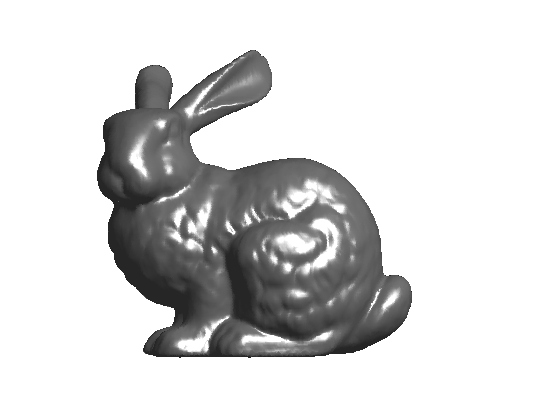Pick a first point.

landmarks = ;


Compute the geodesic distance to this point.

[D,Z,Q] = perform_fast_marching_mesh(vertex, faces, landmarks);


Display the geodesic distance to the point.

clf; hold on;
options.face_vertex_color = mod( 20*D/max(D),1 );
plot_mesh(vertex,faces, options);
colormap jet(256);
h = plot3(vertex(1,landmarks), vertex(2,landmarks), vertex(3,landmarks), 'r.');
set(h, 'MarkerSize', 20);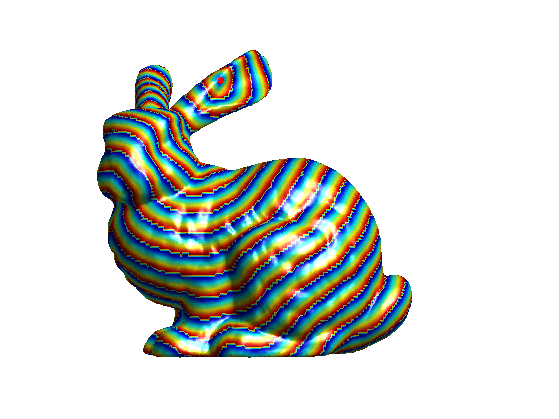Select as the next sampling point the farthest point.

[tmp,landmarks(end+1)] = max(D);


Update the distance map using a local propagation.

options.constraint_map = D;
[D1,Z,Q] = perform_fast_marching_mesh(vertex, faces, landmarks,options);
D = min(D,D1);


Display the update distance map.

clf; hold on;
options.face_vertex_color = mod( 20*D/max(D),1 );
plot_mesh(vertex,faces, options);
colormap jet(256);
h = plot3(vertex(1,landmarks), vertex(2,landmarks), vertex(3,landmarks), 'r.');
set(h, 'MarkerSize', 20);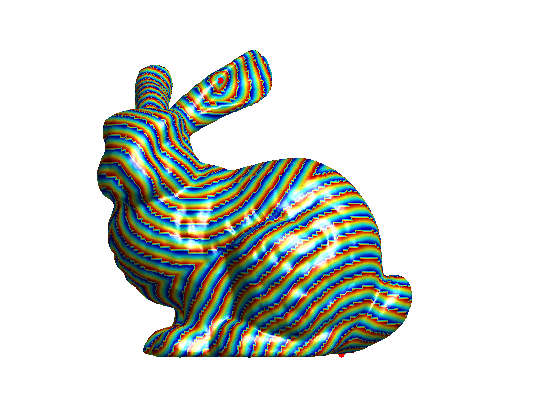Exercice 1: (check the solution) Perform the farthest point sampling of m=500 points.

exo1;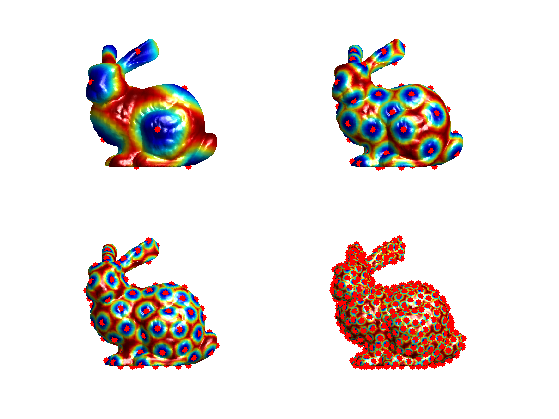## Geodesic Delaunay Triangulation

An intrinsic triangulation of the point is obtained using the geodesic Delaunay triangulation.

Compute the voronoi map Q of the segmentation.

[D,Z,Q] = perform_fast_marching_mesh(vertex, faces, landmarks);


Display the update distance map.

[B,I,J] = unique(Q);
v = randperm(m)'; J = v(J);
clf; hold on;
options.face_vertex_color = J;
plot_mesh(vertex,faces, options);
colormap jet(256);
h = plot3(vertex(1,landmarks), vertex(2,landmarks), vertex(3,landmarks), 'k.');
set(h, 'MarkerSize', 15);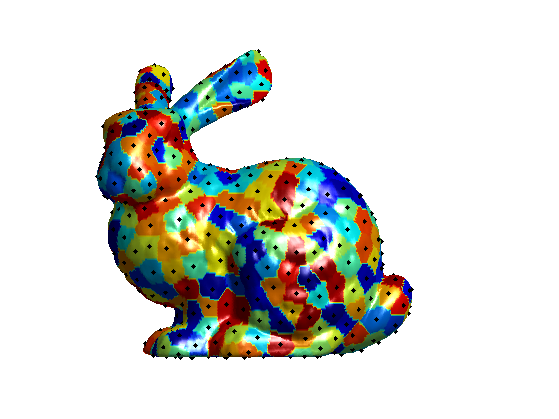Count the number d(i) of different voronoi indexes for each face i.

V = Q(faces); V = sort(V,1);
V = unique(V', 'rows')';
d = 1 + (V(1,:)~=V(2,:)) + (V(2,:)~=V(3,:));


Select the faces with 3 different indexe, they corresponds to geodesic Delaunay faces.

I = find(d==3); I = sort(I);


Build the Delaunay faces set.

z = zeros(n,1);
z(landmarks) = (1:m)';
facesV = z(V(:,I));


Position of the vertices of the subsampled mesh.

vertexV = vertex(:,landmarks);


Re-orient the faces so that they point outward of the mesh.

options.method = 'slow';
options.verb = 0;
facesV = perform_faces_reorientation(vertexV,facesV, options);


Display the sub-sampled mesh.

clf;
options.face_vertex_color = [];
plot_mesh(vertexV,facesV, options);
shading faceted;## Spacially Varying Remeshing

It is possible to seed more point on a given part of the mesh.

Create a density function by designing an isotropic metric. Here we use a metric that is slower in the left part.

W = ones(n,1);
W(vertex(1,:)<median(vertex(1,:))) = .4;
options.W = W;


Display the speed function.

clf;
hold on;
options.face_vertex_color = W;
plot_mesh(vertex,faces, options);
colormap jet(256);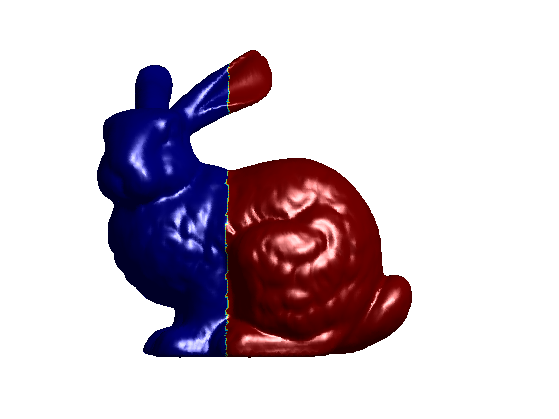Perform front propagation using this speed function.

landmarks = ;
options.constraint_map = [];
[D,Z,Q] = perform_fast_marching_mesh(vertex, faces, landmarks, options);


Display the distance map.

clf;
hold on;
options.face_vertex_color = mod( 20*D/max(D),1 );
plot_mesh(vertex,faces, options);
colormap jet(256);
h = plot3(vertex(1,landmarks), vertex(2,landmarks), vertex(3,landmarks), 'r.');
set(h, 'MarkerSize', 20);Exercice 2: (check the solution) Perform a spacially adative remeshing.

exo2;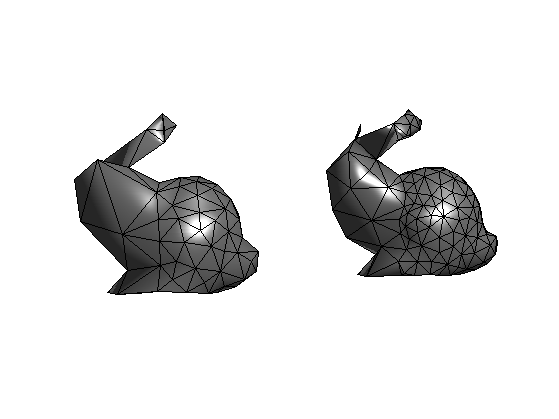## Feature Sensitive Remeshing

A better remeshing quality is obtained by sampling more densly sharp features. This is achieved using a spatially varying metric, so that the front propagate slowly near regions of high curvature.

Compute the curvature of the mesh.

[Umin,Umax,Cmin,Cmax,Cmean,Cgauss,Normal] = compute_curvature(vertex,faces,options);


Compute the total curvature.

C = abs(Cmin)+abs(Cmax);


Display it.

clf;
hold on;
options.face_vertex_color = min(C,.1);
plot_mesh(vertex,faces, options);
colormap jet(256);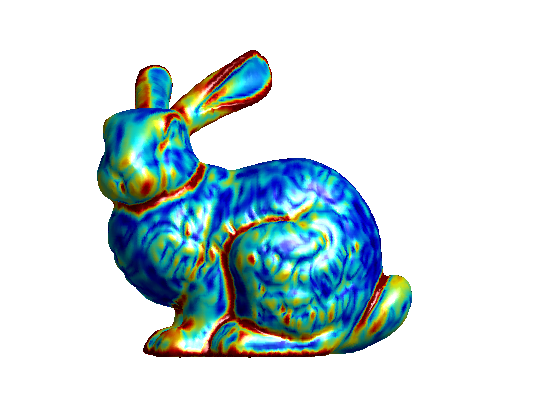Exercice 3: (check the solution) Design a metric W so that the sampling is densed in area where C is large.

exo3;Exercice 4: (check the solution) Use such a metric to perform feature sensitive remeshing. Tune the metric to reduce as much as possible the Hausdorff approximation error.

exo4;